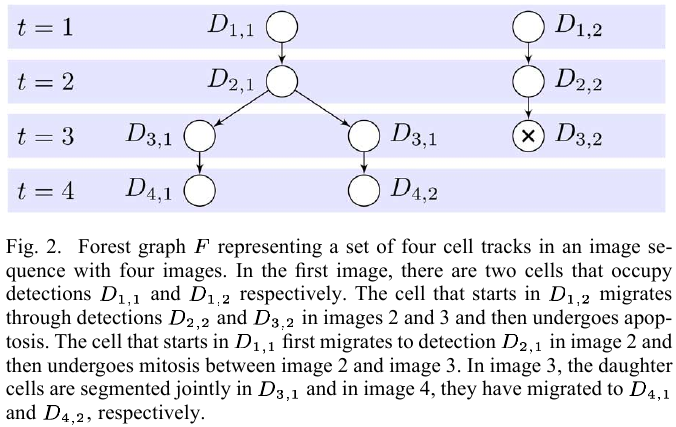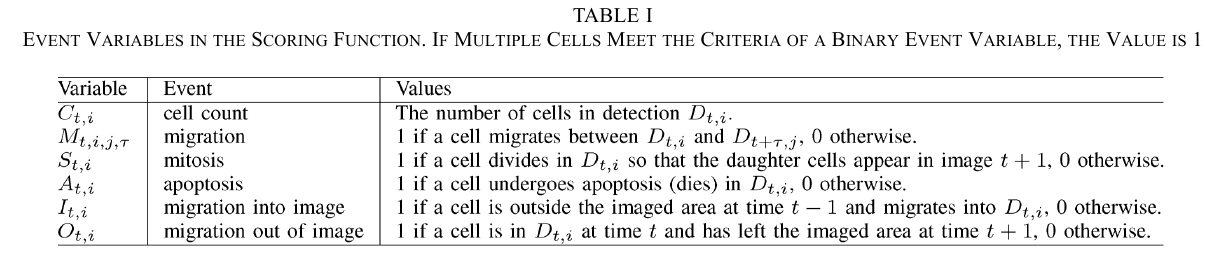# Global Tracking via the Viterbi Algorithm

##### Posted on Aug 27, 2020
Tags: Object Tracking

In cell and developmental biology, different cell types are studied either

• in cell cultures (in vitro)
• in live organisms or embryos (in vivo)
• in live tissue (in situ)

Time-lapse microscopy can be used to characterize and quantify many different aspects of cell behavior, such as proliferation (增殖), mitosis (cell division), apoptosis (cell death), migration, and morphology.

Cell tracking algorithms are normally classified into

• model evolution algorithms, where mathematical models of the cells are propagated in time
• tracking by detection, where the tracking problem is separated into finding the outlines of the cells (segmentation) and linking the detected outlines into tracks (track linking, data association, or tracking)

The main challenge of the track linking problem is to perform data association despite errors in the segmentation.

## Problem Statement

Assume that the segmentation algorithms outputs a set of detections $\cal D={D_{t,i}}_{t=1:T,i=1:N_t}$.For every set of proposed tracks $F$, there is a unique set of assignments to the event variables $\cE(F)={\cE_m(F)}_{m=1:\vert\cE\vert}$, then define a score $g(F)$ for the set of tracks $F$, as the sum of the logarithmic probabilities of the assignments to the individual event variables, i.e.,

$g(F) = \sum_{m=1}^{\vert \cE\vert}\log \Pr(\cE_m = \cE_m(F))\,.$The scoring function is memoryless in the sense that the score associated with an event in a cell track does not depend on prior events in the track. This is equivalent to a Markov property saying that the event following the current time-point is independent of prior states of the cell, given the current state of the cell.

• the change in the score of $F$, that occurs when a track is added, can be computed as the sum of the arc utilities along the corresponding path through the state space diagram.